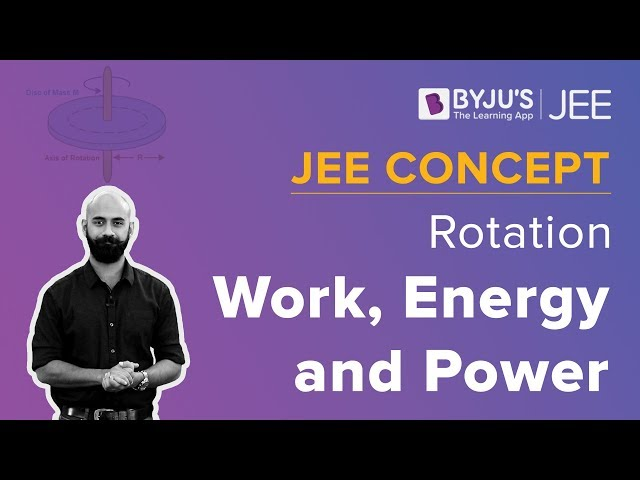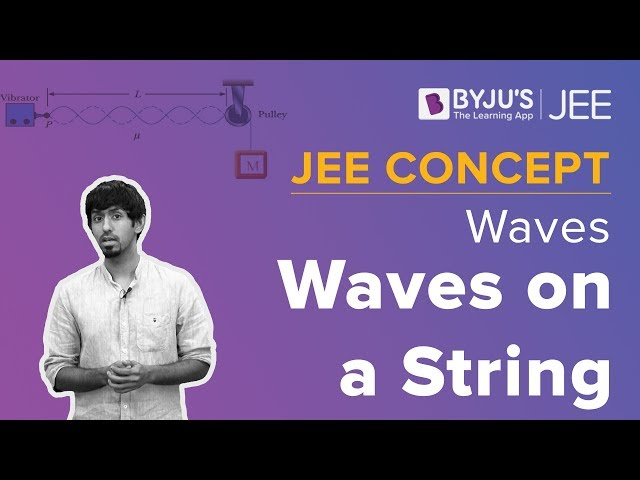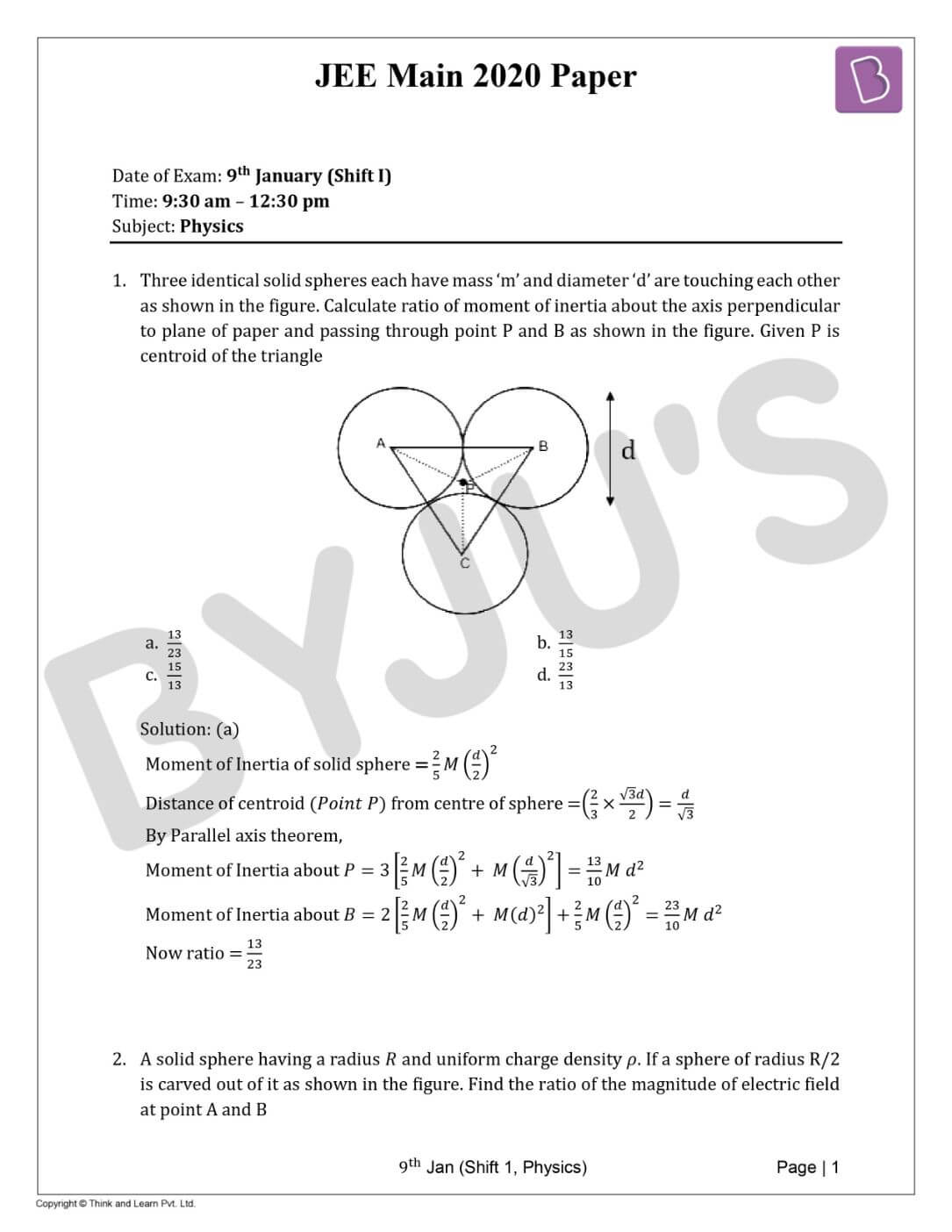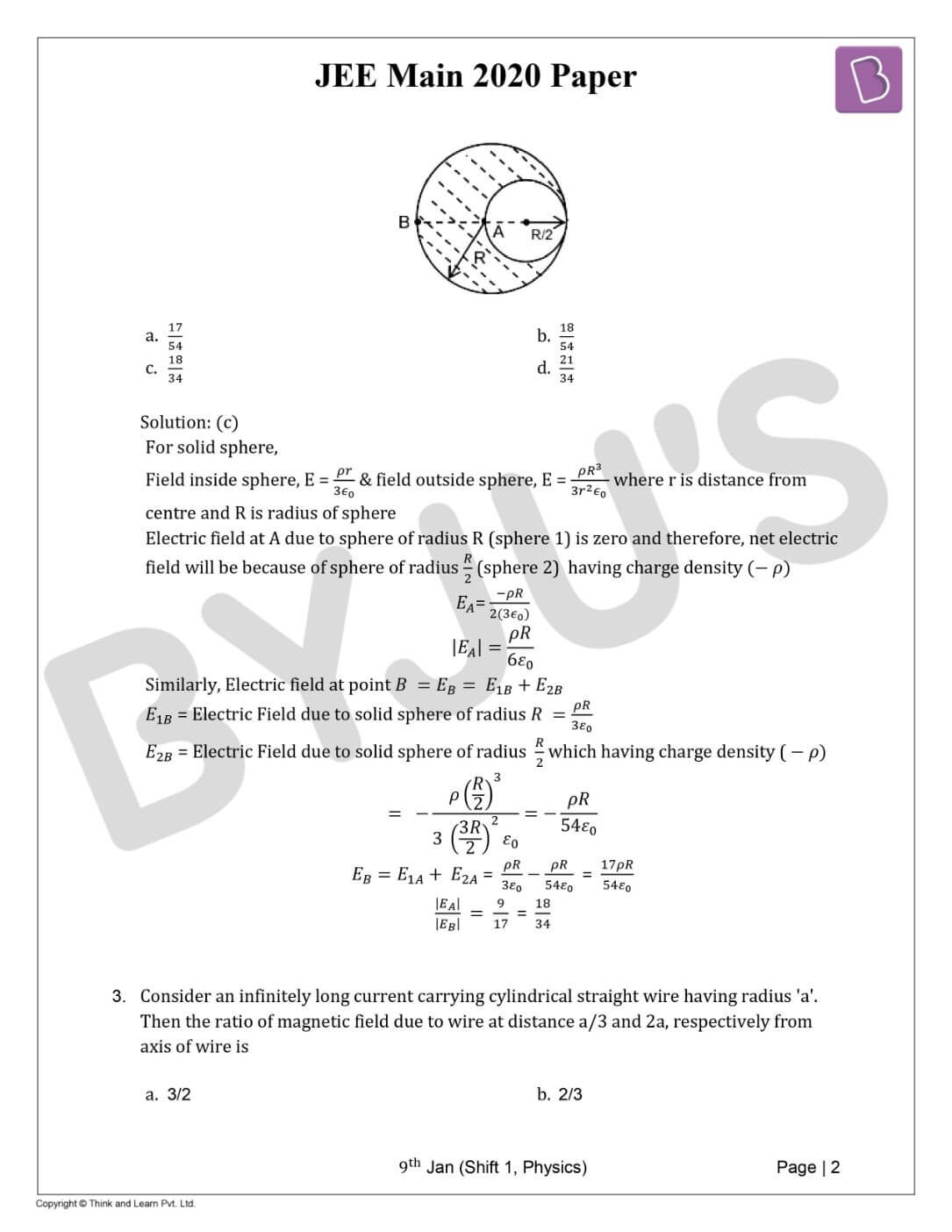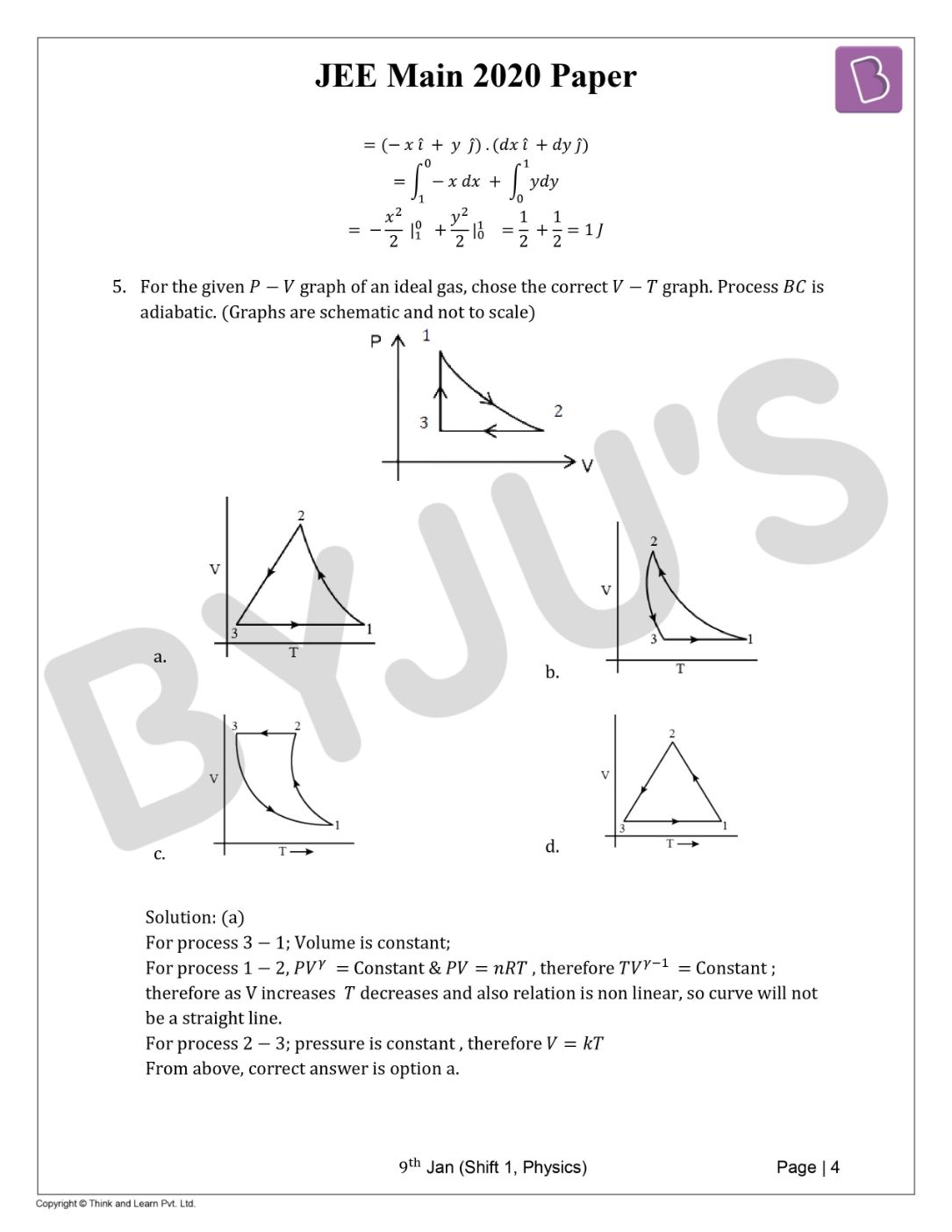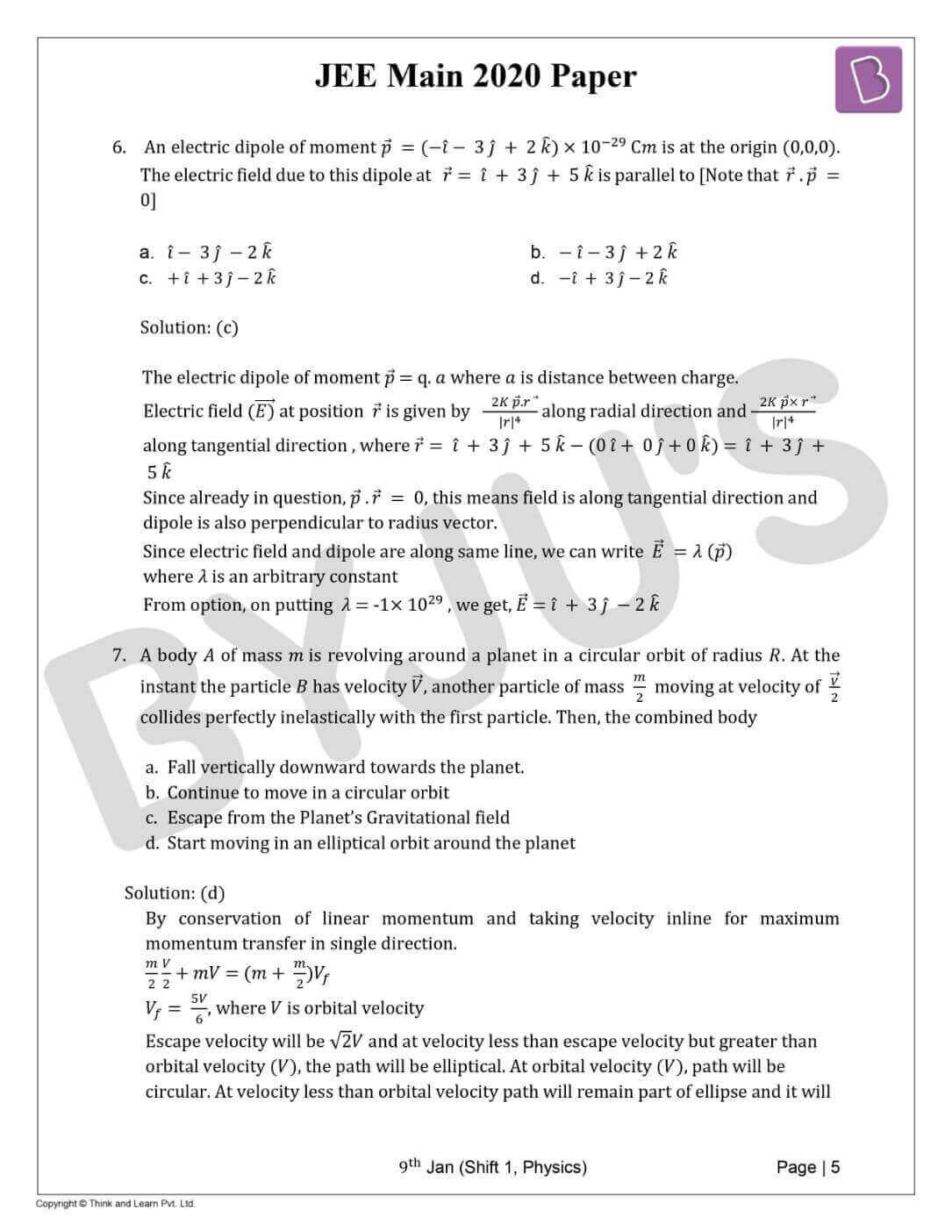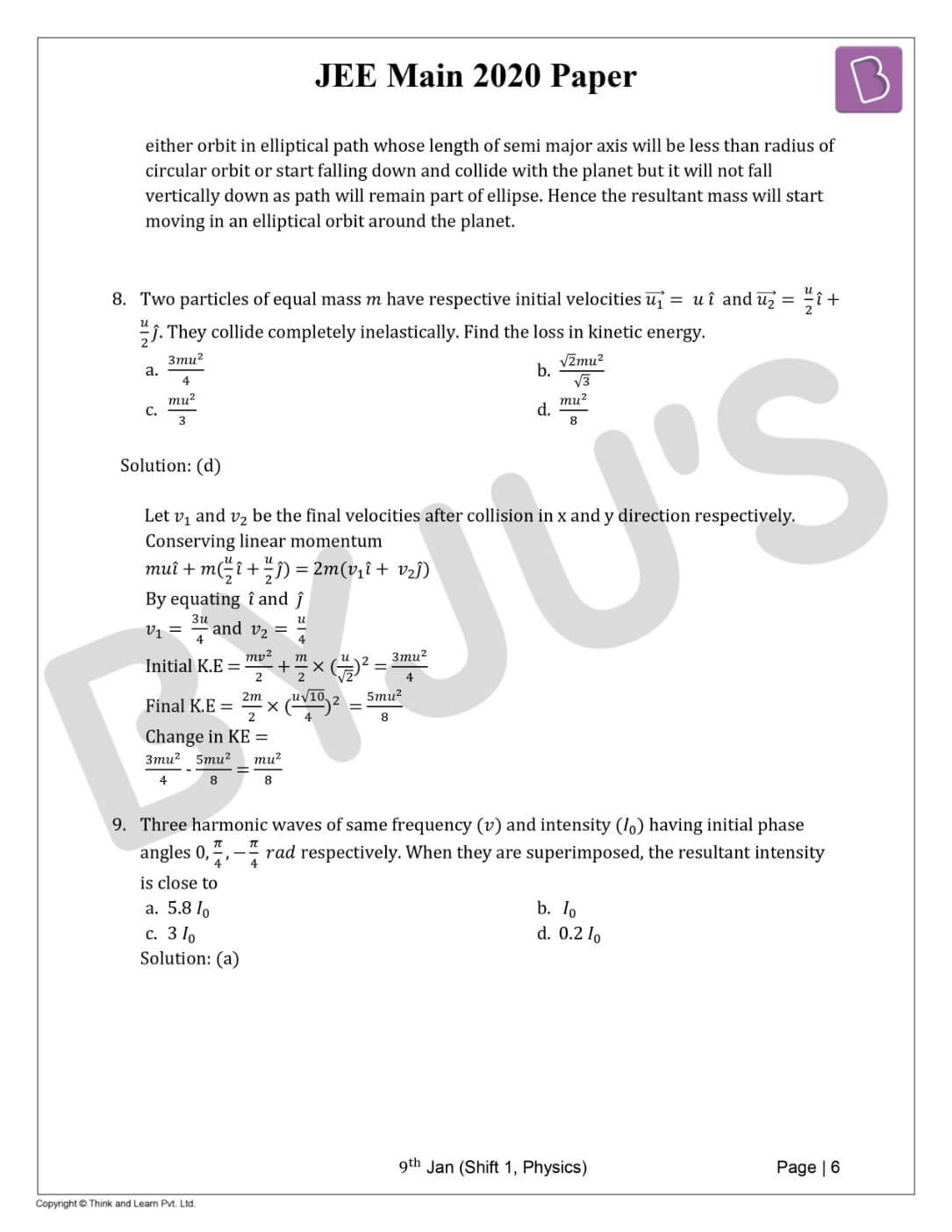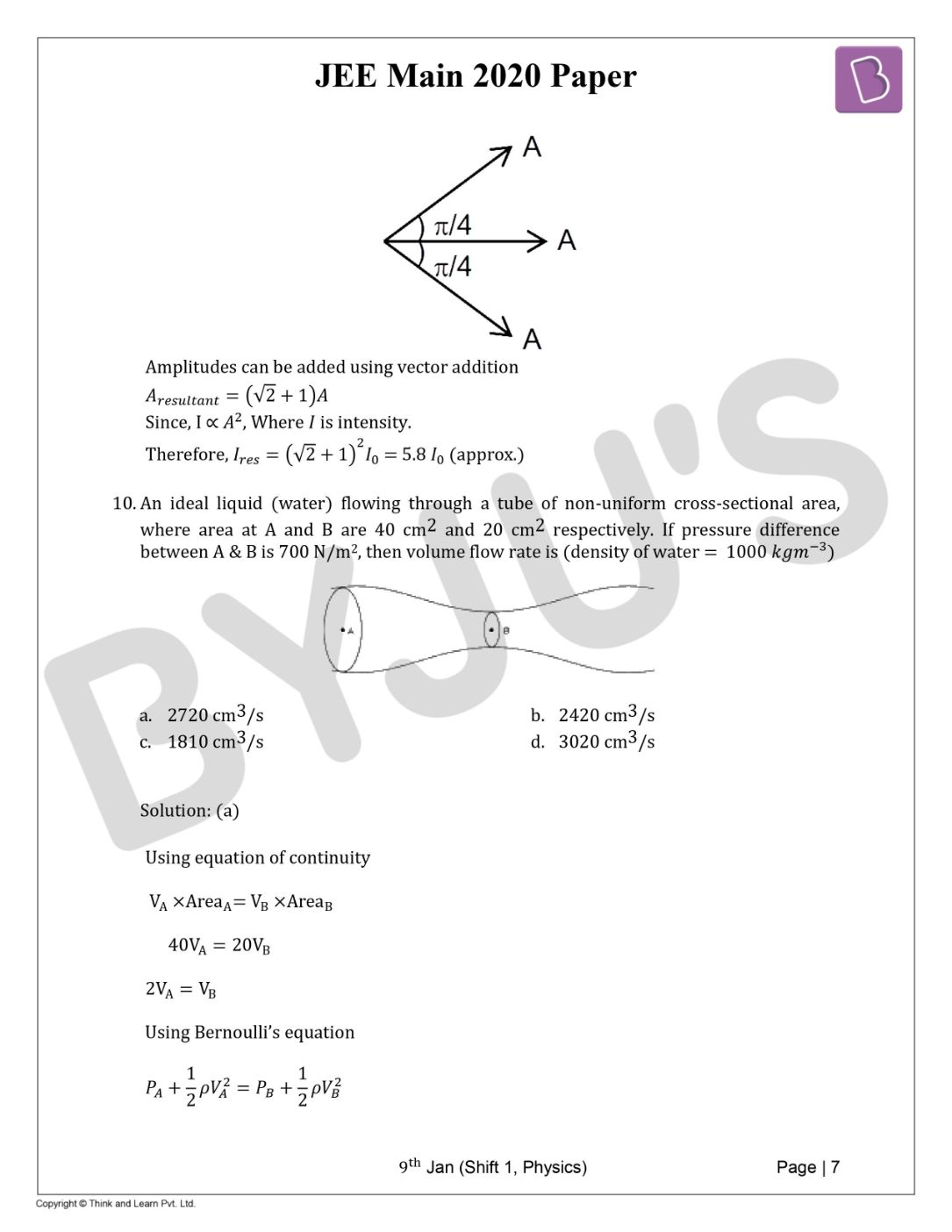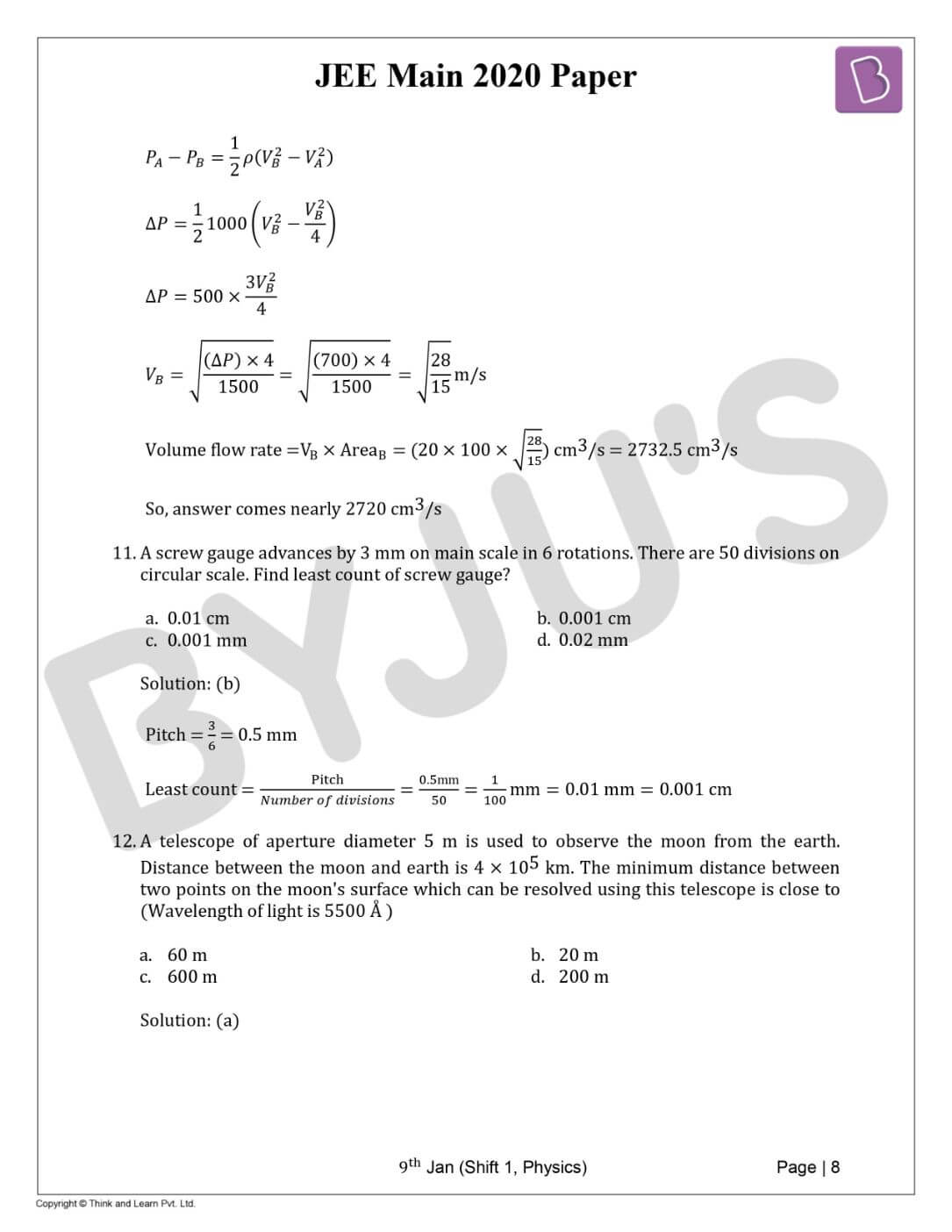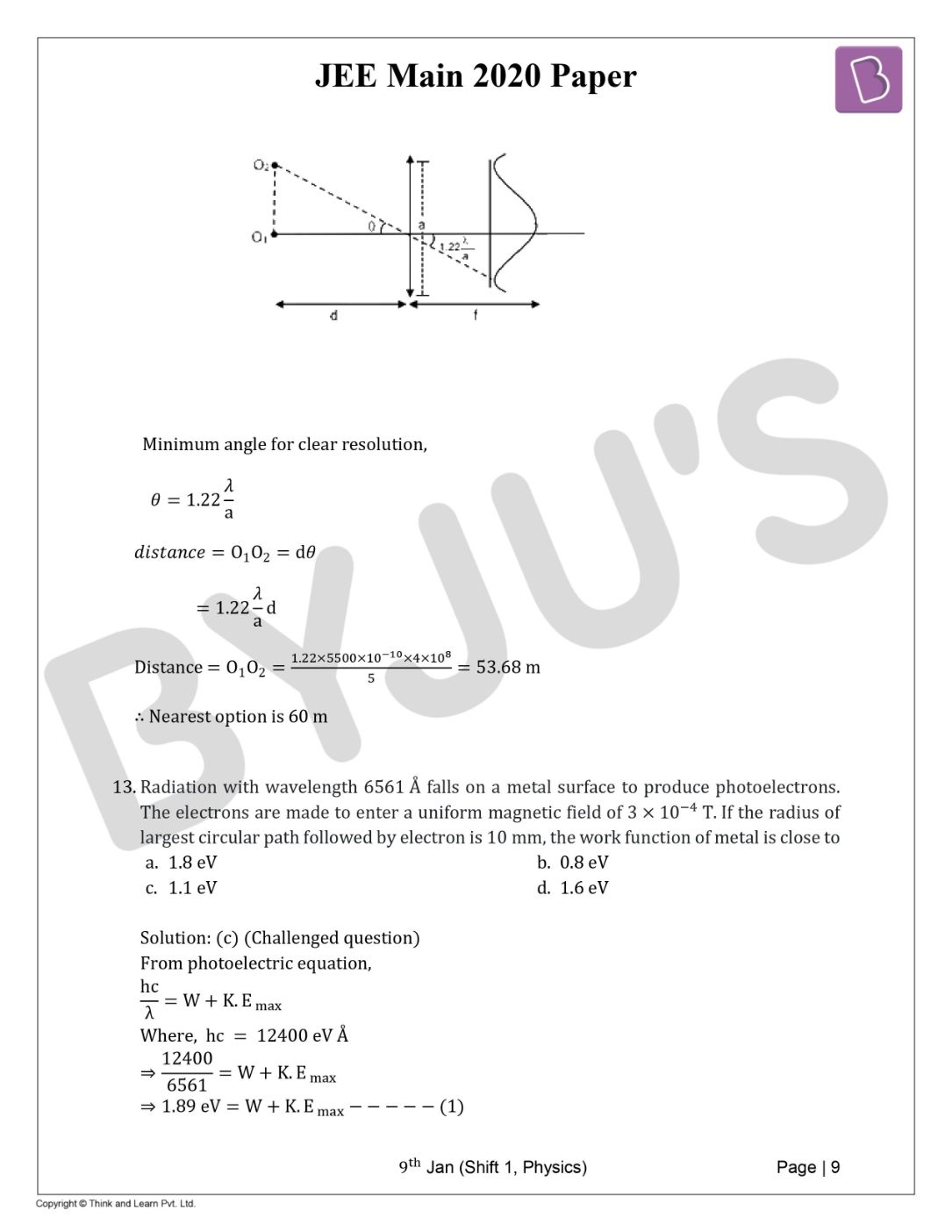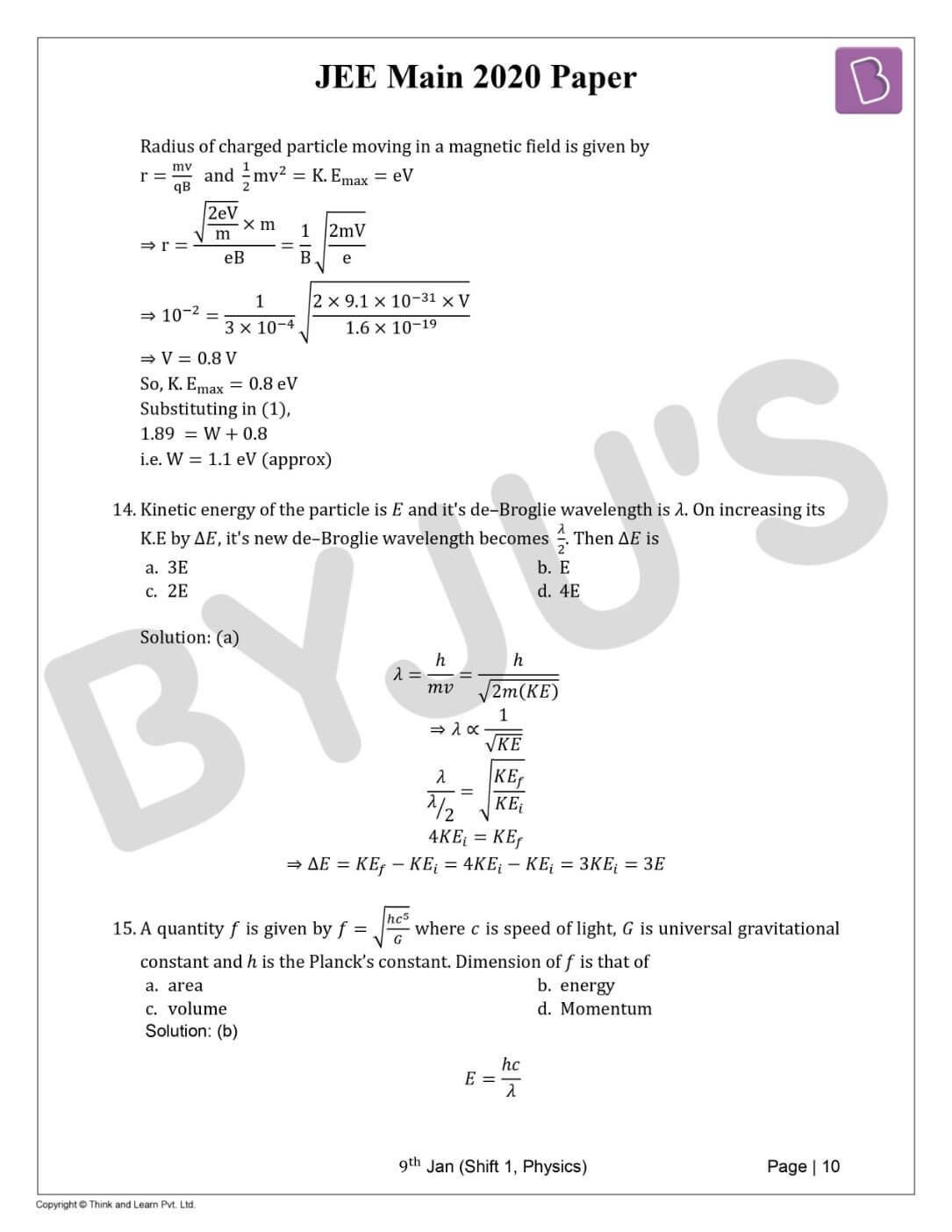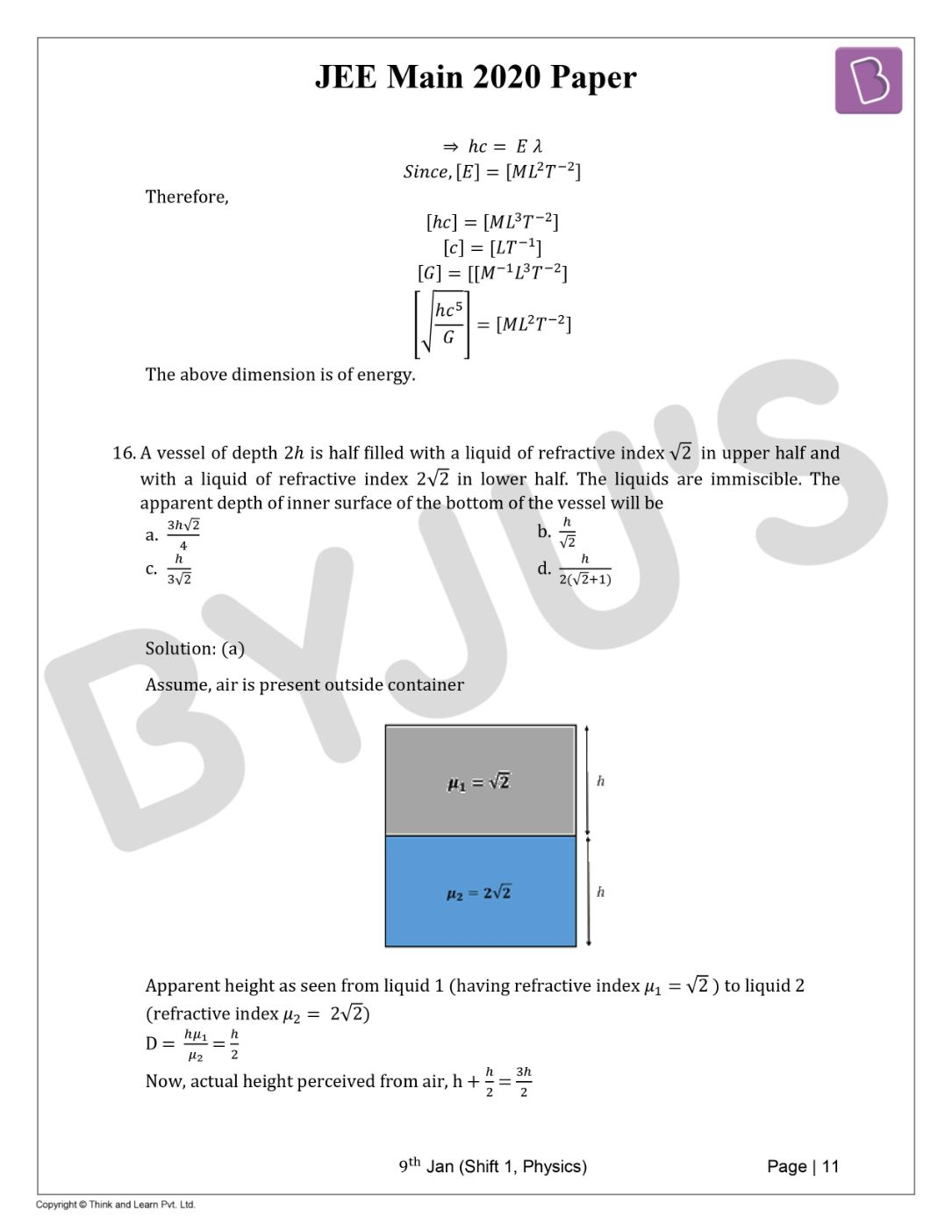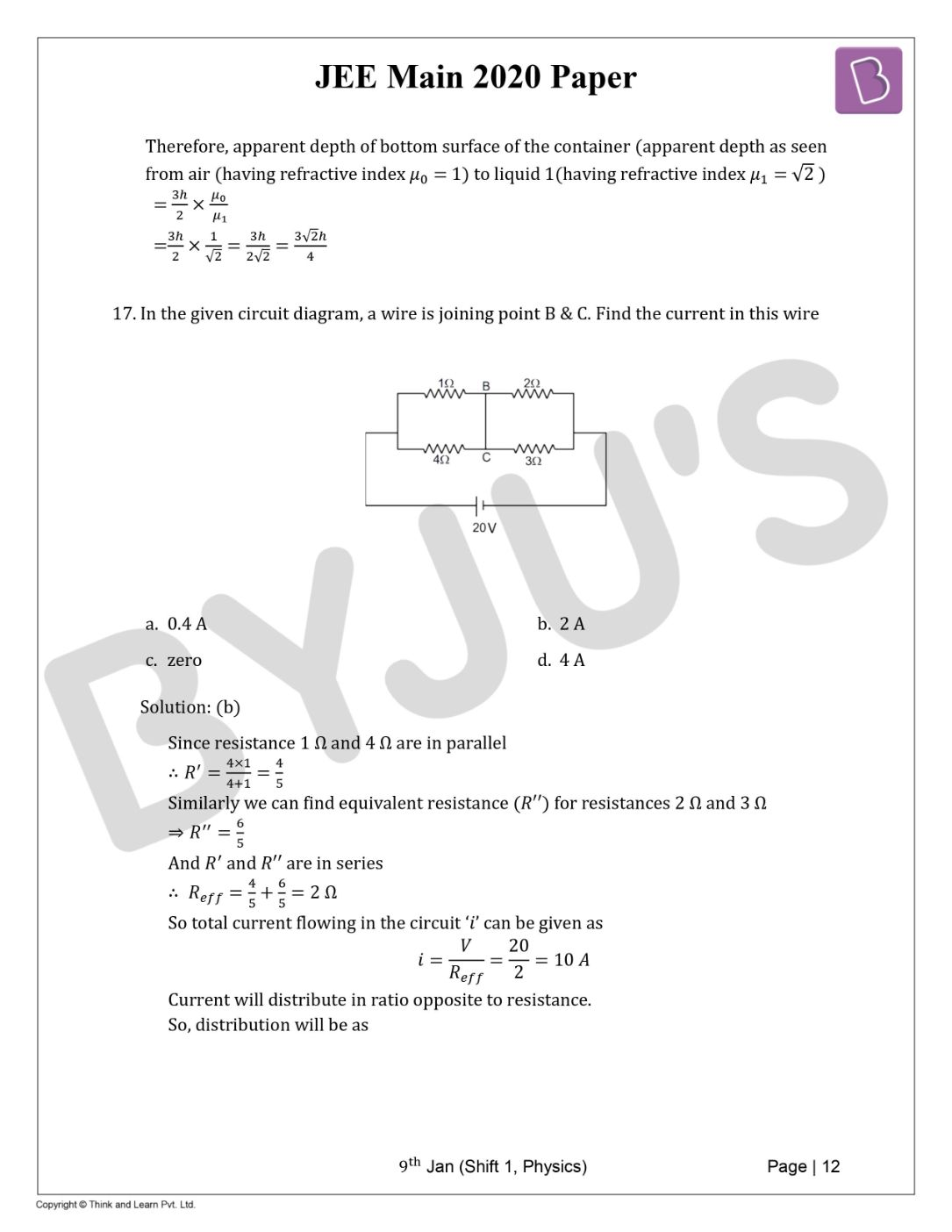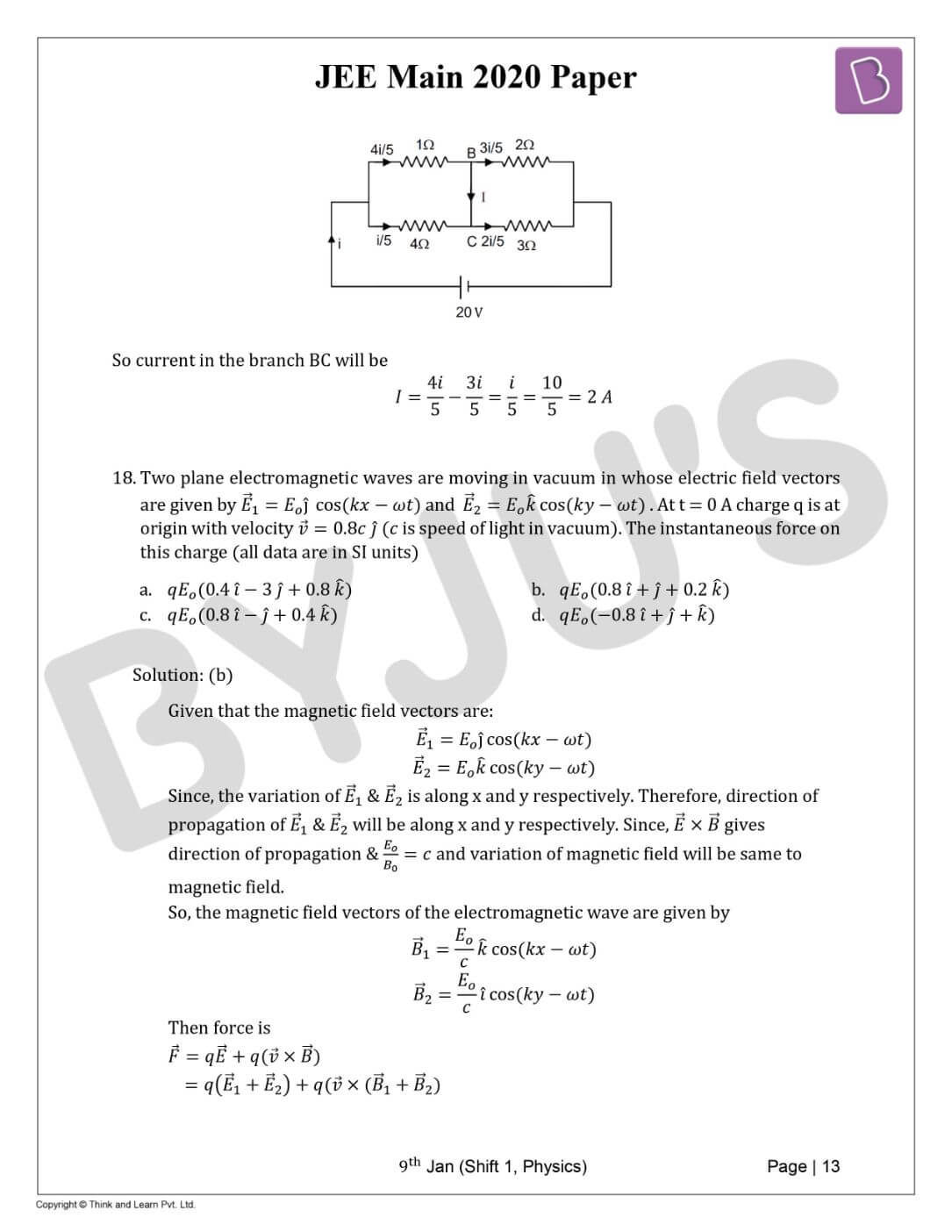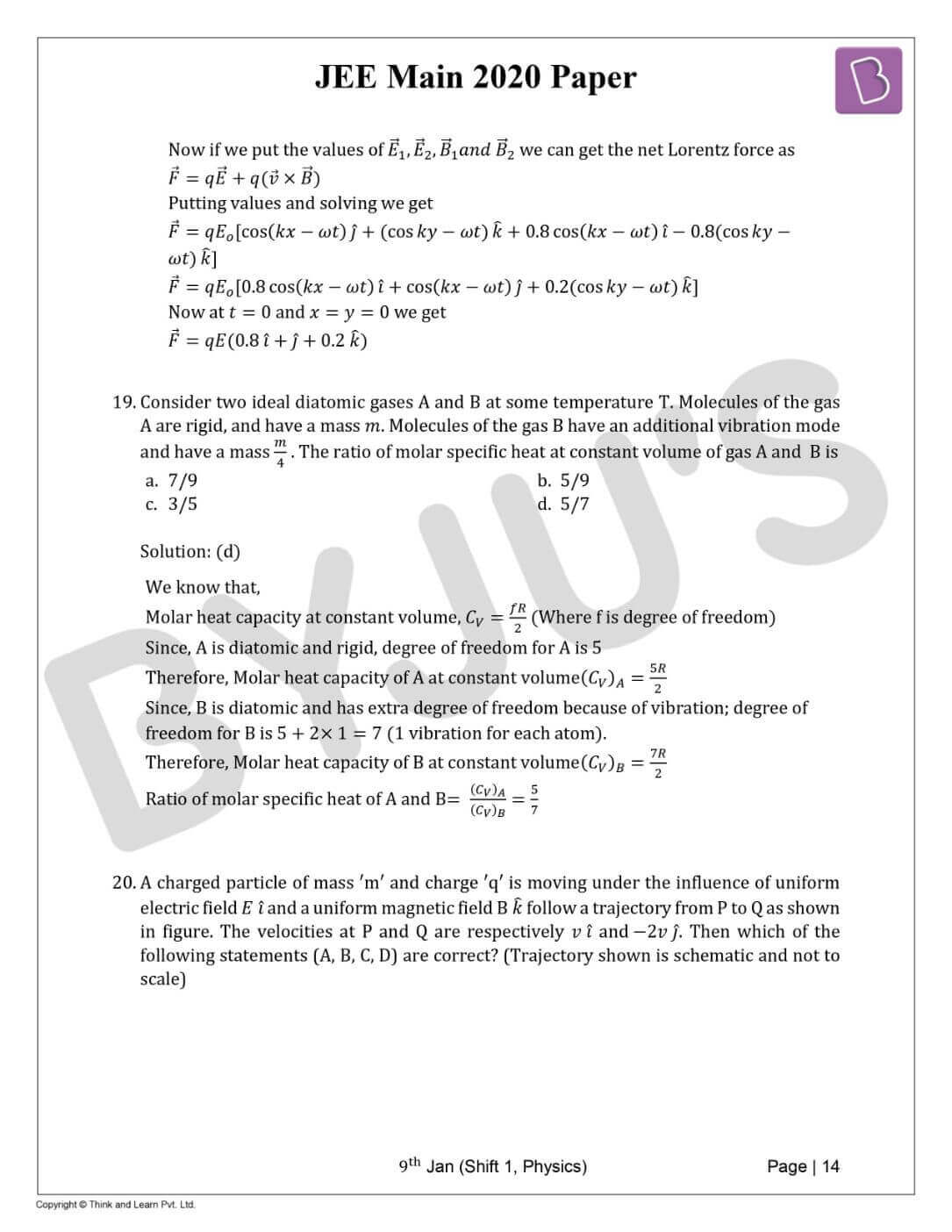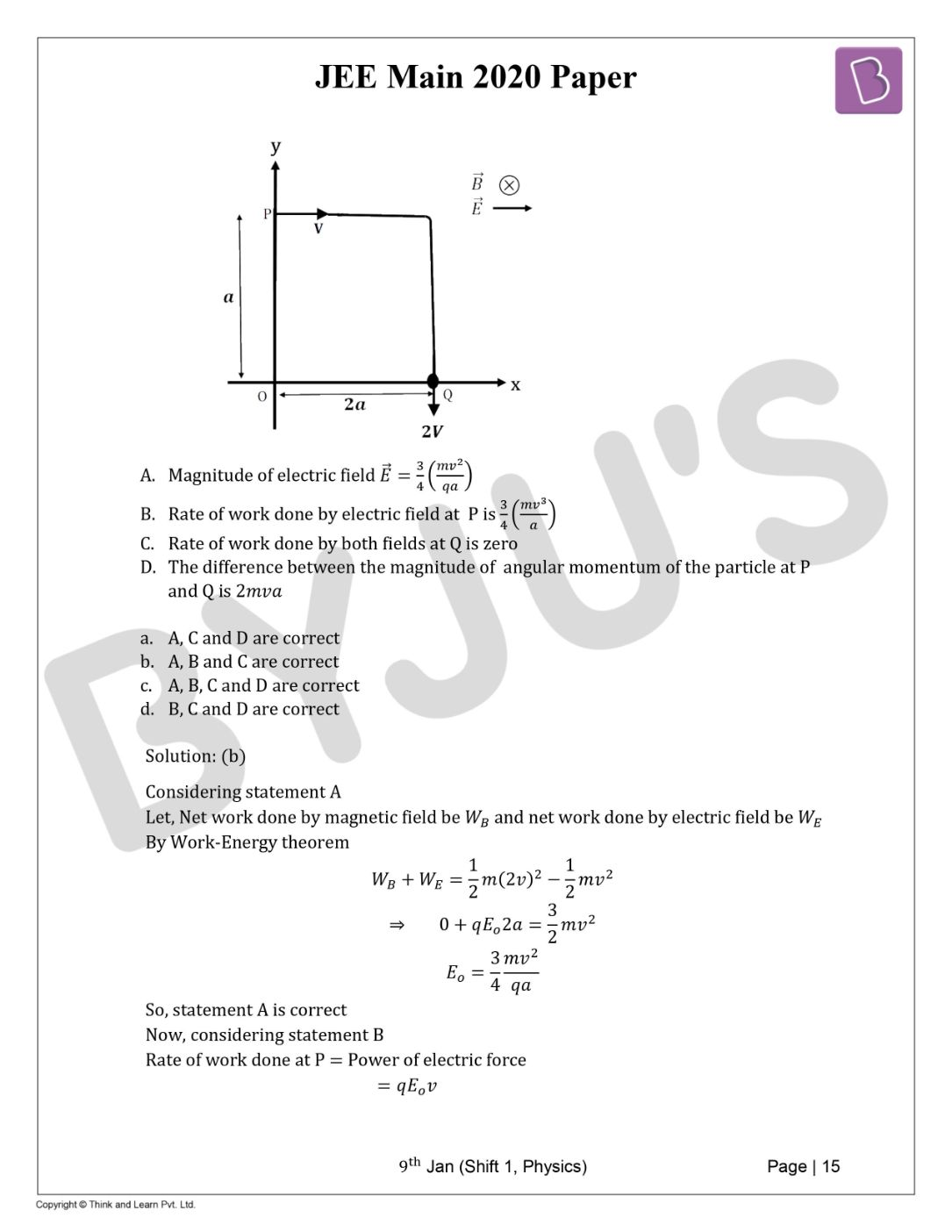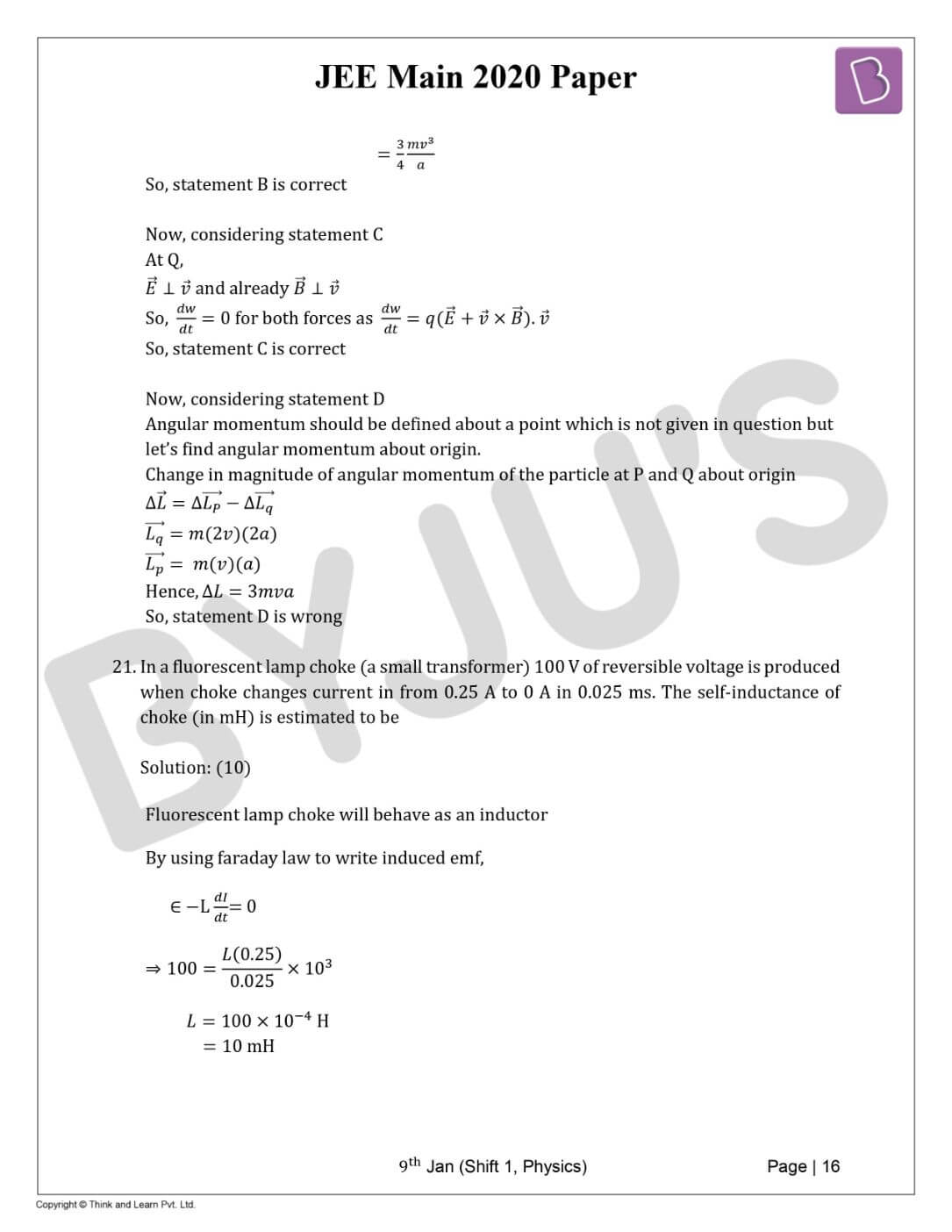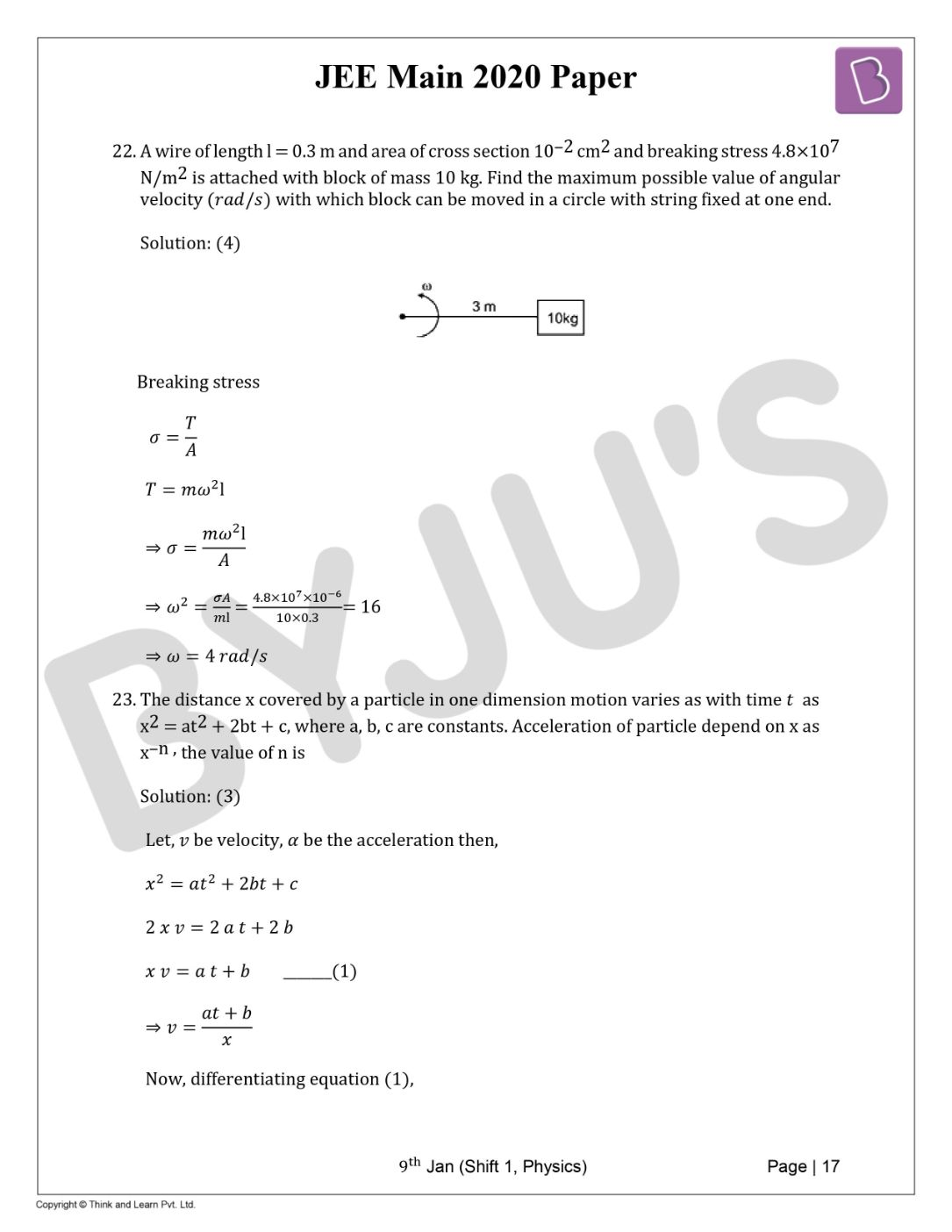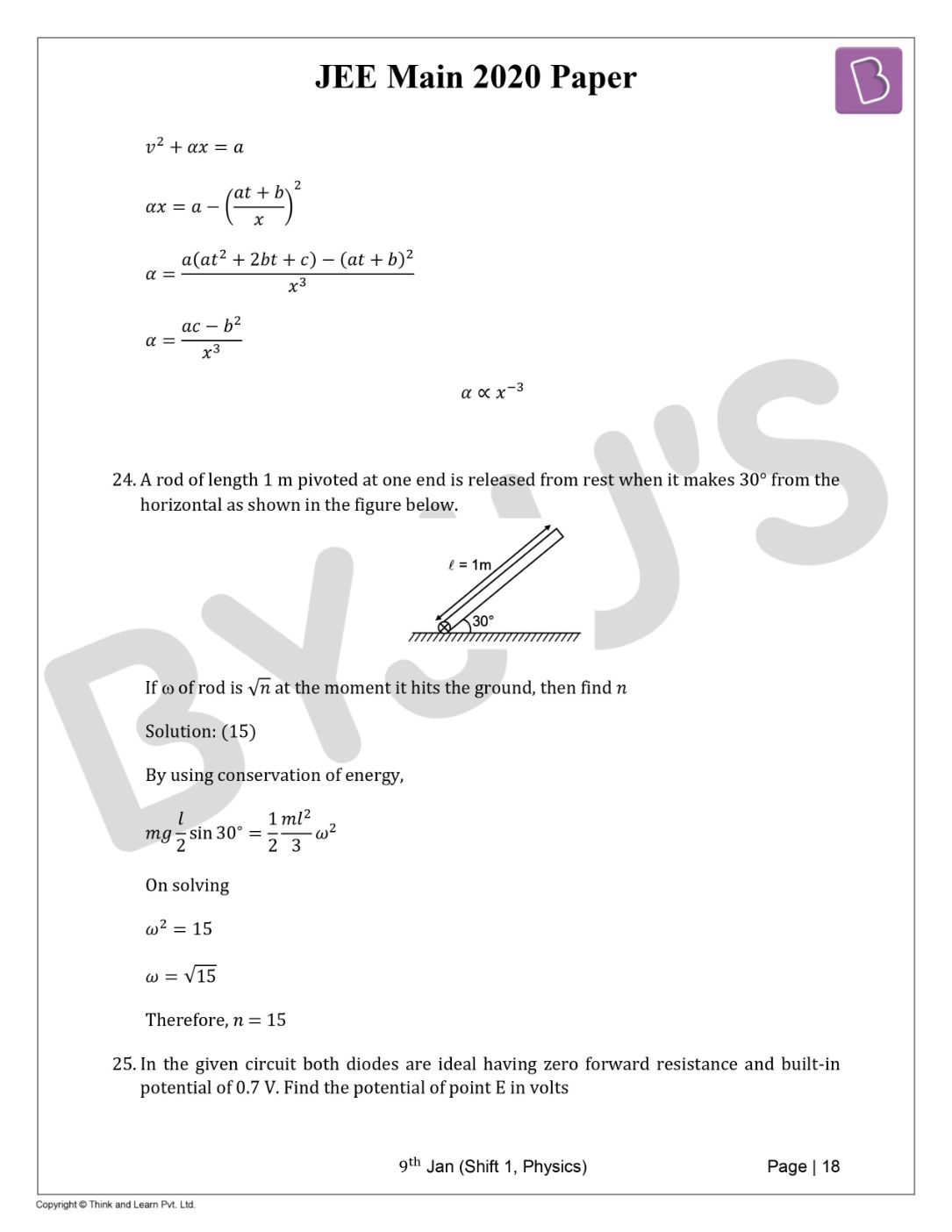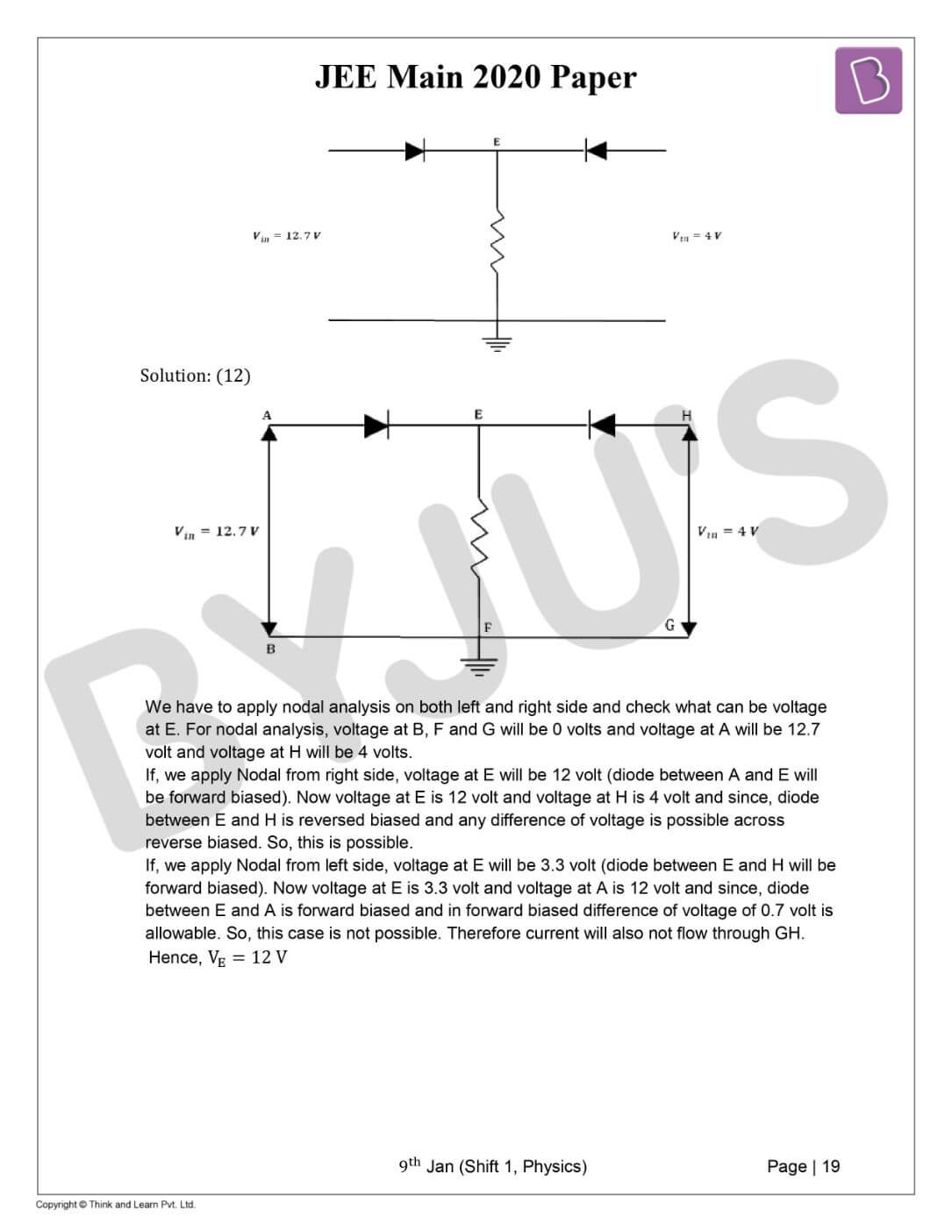Win up to 100% scholarship on Aakash BYJU'S JEE/NEET courses with ABNAT Win up to 100% scholarship on Aakash BYJU'S JEE/NEET courses with ABNAT

# JEE Main 2020 Physics Paper With Solutions Jan 9 Shift 1

JEE Main 2020 9th Jan shift 1 solved Physics paper consists of accurate solutions, prepared by our subject experts. These step by step solutions will help students to understand the problems easily. Students are recommended to practise these solutions to self analyse their preparation level and thus find out their weaker areas and concentrate more on those topics. Students can easily access these solutions from our website and download them in PDF format for free.
January 9 Shift 1 – Physics
Question 1. Three identical solid spheres each have mass ‘m’ and diameter ‘d’ are touching each other as shown in the figure. Calculate ratio of moment of inertia about the axis perpendicular to plane of paper and passing through point P and B as shown in the figure. Given P is centroid of the triangle.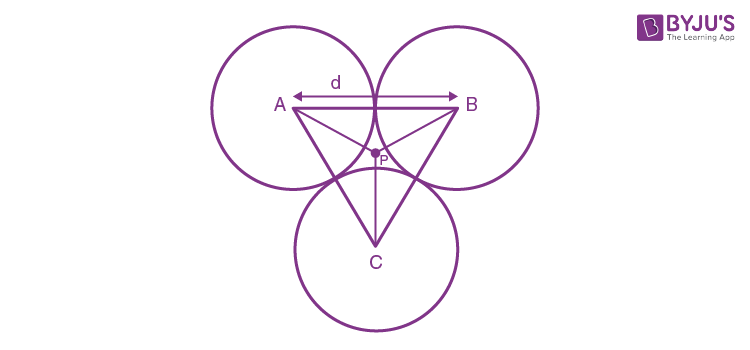a) 13/23
b) 13/15
c) 15/13
d) 23/13

Distance of centroid from (point P) centre of sphere = (2/3)×(v3d/2) = d/v3

By Parallel axis theorem,

Moment of Inertia about P = 3[ (2/5)M(d/2)2 + M(d/v3)2] = (13/10) Md2

Moment of Inertia about B = 2[ (2/5)M(d/2)2 + M(d)2] + (2/5)M(d/2)2 = (23/10)Md2

Now ratio = 13/23

Question 2. A solid sphere having a radius R and uniform charge density ?. If a sphere of radius R/2 is carved out of it as shown in the figure. Find the ratio of the magnitude of electric field at point A and B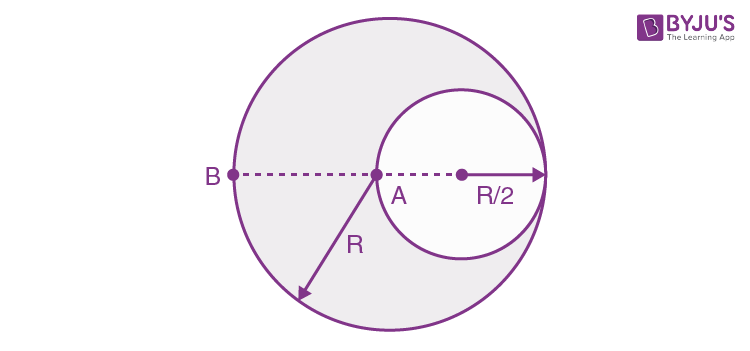a) 17/54
b) 18/54
c) 18/34
d) 21/34

For solid sphere,

Field inside sphere, E = ?r/3e0

And field outside sphere, E = ?r3/3r2e0

where r is distance from centre and R is radius of sphere.

Electric field at A due to sphere of radius R (sphere 1) is zero and therefore, net electric field will be because of sphere of radius R/2 (sphere 2) having charge density (-?)

EA = – ?R/2(3 e0)

|EA| = ?R/6 e0

Similarly, Electric field at point B = EB = E1B + E2B

E1B = Electric Field due to solid sphere of radius R = ?r/3e0

E2B = Electric Field due to solid sphere of radius R/2 which having charge density (-?)

=

$$\begin{array}{l}-\frac{\rho (\frac{R }{2})^{3}}{3(\frac{3R}{2})^{2}\varepsilon _{0}}\end{array}$$

= -?R/54e0

EB = E1A + E2A = (?R/3e0) – (?R/54e0)

= 17 ?R/54e0

$$\begin{array}{l}\frac{\left | E_{A} \right |}{\left | E_{B} \right |} = \frac{9}{17} =\frac{18}{34}\end{array}$$

Question 3. Consider an infinitely long current carrying cylindrical straight wire having radius ‘a’. Then the ratio of magnetic field due to wire at distance a/3 and 2a, respectively from axis of wire is
a) 3/2
b) 2/3
c) 2
d) 1/2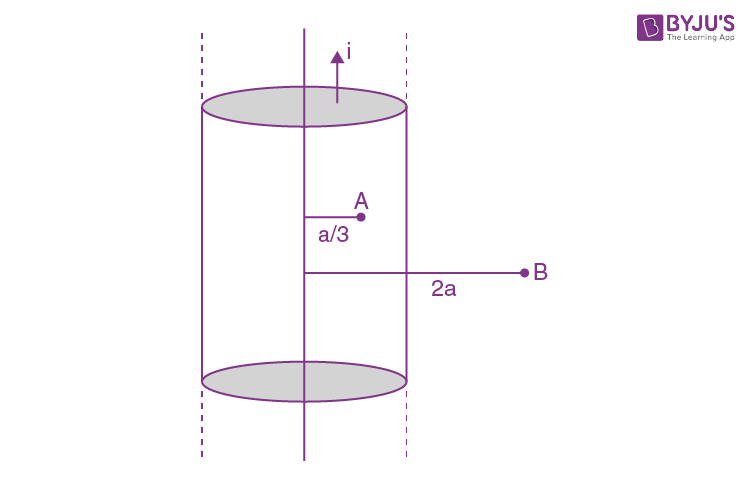BA =

$$\begin{array}{l}\frac{\mu _{0}ir}{2\pi a^{2}} = \frac{\frac{\mu _{0}ia}{3}}{2\pi a^{2}}\end{array}$$
$$\begin{array}{l}= \frac{{\mu _{0}ia}}{6\pi a^{2}} = \frac{\mu _{0}i}{6\pi a}\end{array}$$

BB = µ0i(2a)/2p(2a)2 = µ0i /4pa

BA/BB = 4/6 = 2/3

Question 4. Particle moves from point ?? to point ?? along the line shown in figure under the action of force
$$\begin{array}{l}\vec{F} = -x\hat{i}+ y\hat{i}\end{array}$$
. Determine the work done on the particle by
$$\begin{array}{l}\vec{F}\end{array}$$
in moving the particle from point A to point B (all quantities are in SI units)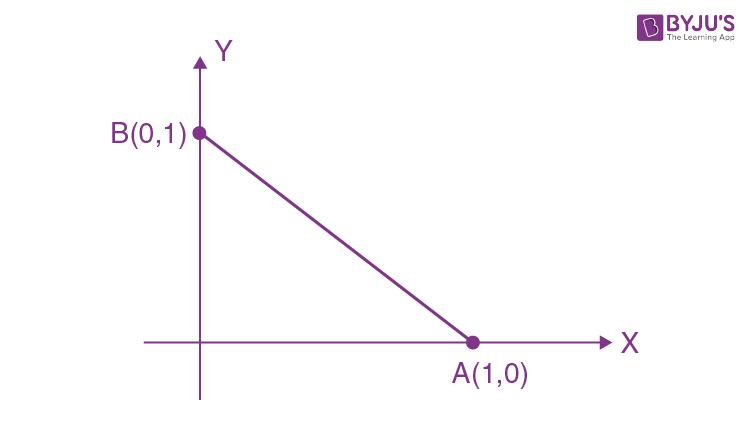a) 1 J
b) 1/2 J
c) 2 J
d) 3/2 J

$$\begin{array}{l}d\vec{s} = (dx \; \hat{i} + dy\; \hat{j})\end{array}$$
$$\begin{array}{l}= (-x\hat{i}+ y\hat{j}). (dx\hat{i}+ dy\hat{j})\end{array}$$
$$\begin{array}{l}=\int_{1}^{0}-x\: dx+ \int_{0}^{1}y\: dy\end{array}$$

= ½ + ½

= 1 J

Question 5. For the given P-V graph of an ideal gas, chose the correct V-T graph. Process BC is adiabatic. (Graphs are schematic and not to scale).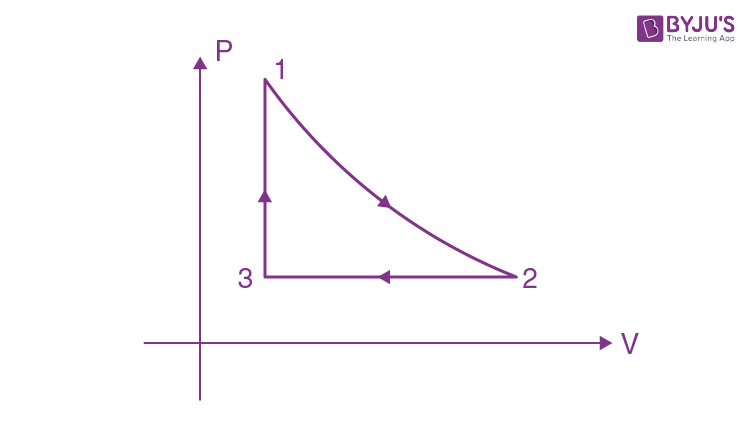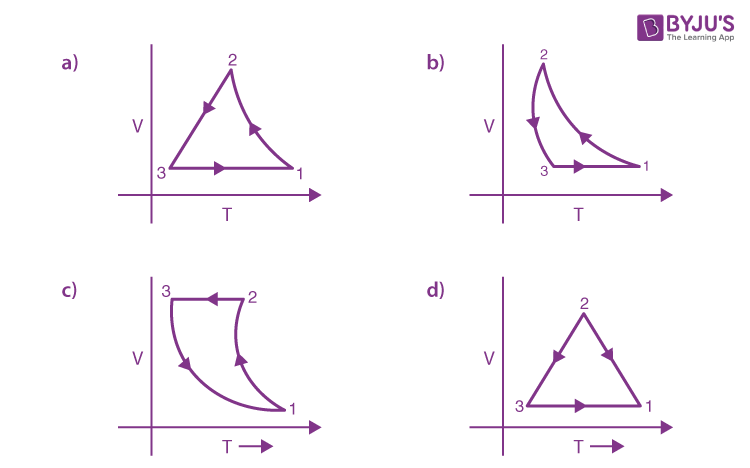For process 3 – 1; Volume is constant;

For process 1 – 2, PV? Constant , and PV = nRT therefore TV?-1 = Constant ;

therefore as V increases T decreases and also relation is non linear, so curve will not be a straight line.

For process 2-3; pressure is constant , therefore V = kT

From above, correct answer is option a.

Question 6.An electric dipole of moment
$$\begin{array}{l}\vec{p} = (-\hat{i}-3\hat{j}+2\hat{k})\times 10^{-29}Cm\end{array}$$
is at the origin (0,0,0). The electric field due to this dipole at
$$\begin{array}{l}\vec{r} = (\hat{i}+3\hat{j}+5\hat{k})\end{array}$$
is parallel to [Note that
$$\begin{array}{l}\vec{r} .\vec{p} = 0\end{array}$$
]

a) i -3j-2k
b) -i-3j+2k
c) i+3j-2k
d) -i+3j-2k

The electric dipole of moment p = q.a where a is distance between charge.

Electric field E at position r is given by

$$\begin{array}{l}\frac{2K\vec{p} .\vec{r} }{\left | r \right |^{4}}\end{array}$$
$$\begin{array}{l}\frac{2K\vec{p} \times \vec{r} }{\left | r \right |^{4}}\end{array}$$

along tangential direction , where

$$\begin{array}{l}\vec{r} =\hat{i}+3\hat{j}+5\hat{k}-(0\hat{i}+0\hat{j}+0\hat{k})= \hat{i}+3\hat{j}+5\hat{k}\end{array}$$

$$\begin{array}{l}\vec{p}.\vec{r} =0\end{array}$$

This means field is along tangential direction and dipole is also perpendicular to radius vector.

Since electric field and dipole are along same line, we can write

$$\begin{array}{l}\vec{E} = \lambda (\vec{p})\end{array}$$
where ? is an arbitrary constant

From option, on putting ? = -1× 1029 , we get,

$$\begin{array}{l}\vec{E} = \hat{i}+3\hat{j}-2\hat{k}\end{array}$$

Question 7. A body A of mass m is revolving around a planet in a circular orbit of radius R. At the instant the particle B has velocity , another particle of mass m/2 moving at velocity of V/2, collides perfectly inelastically with the first particle. Then, the combined body
a) Fall vertically downward towards the planet.
b) Continue to move in a circular orbit
c) Escape from the Planet’s Gravitational field
d) Start moving in an elliptical orbit around the planet

By conservation of linear momentum and taking velocity inline for maximum momentum transfer in single direction.

(m/2)(V/2) + mV = [m + (m/2)]Vf

Vf = 5V/6

Where V is orbital velocity

Escape velocity will be v2V and at velocity less than escape velocity but greater than orbital velocity (V), the path will be elliptical. At orbital velocity (??), path will be circular. At velocity less than orbital velocity path will remain part of ellipse and it will either orbit in elliptical path whose length of semi major axis will be less than radius of circular orbit or start falling down and collide with the planet but it will not fall vertically down as path will remain part of ellipse. Hence the resultant mass will start moving in an elliptical orbit around the planet.

Question 8. Two particles of equal mass m have respective initial velocities
$$\begin{array}{l}\vec{u_{1}} = u\hat{i}\end{array}$$
and
$$\begin{array}{l}\vec{u_{2}} = \frac{u}{2}\hat{i} + \frac{u}{2}\hat{j}\end{array}$$
. They collide completely inelastically. Find the loss in kinetic energy.

a) 3mu2/4
b) v2mu2/v3
c) mu2/3
d) mu2/8

Let v1 and v2 be the final velocities after collision in x and y direction respectively.

Conserving linear momentum

$$\begin{array}{l}mu\hat{i} + m(\frac{u}{2}\hat{i}+\frac{u}{2}\hat{j}) = 2m(v_{1}\hat{i}+v_{2}\hat{j})\end{array}$$

By equating i and j

v1 = 3u/4

v2 = u/4

Initial K.E = (mv2/2) + (m/2)×(u/v2)2 = 3mu2/4

Final K.E = (2m/2)×(uv10/4)2 = 5mu2/8

Change in K.E = (3mu2/4) – (5mu2/8) = mu2/8

Question 9.Three harmonic waves of same frequency (v) and intensity (I0) having initial phase angles 0, p/4, -p/4 rad respectively. When they are superimposed, the resultant intensity is close to;
a) 5.8 I0
b) I0
c) 3 I0
d) 0.2 I0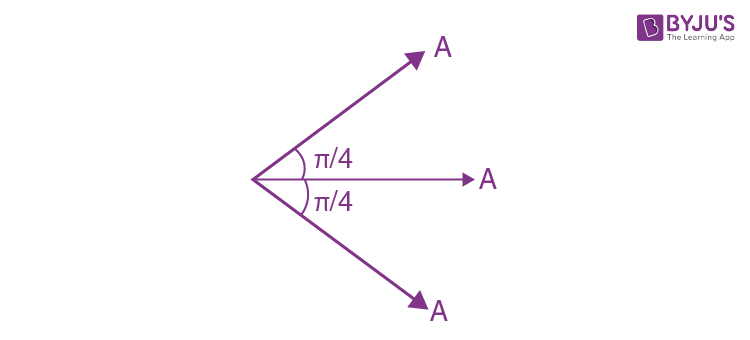Since, I ?A2, Where ?? is intensity.

Therefore, Ires = (v2+1)2 ??0 = 5.8 ??0 (approx.)

Question 10. An ideal liquid (water) flowing through a tube of non-uniform cross-sectional area, where area at A and B are 40 cm2 and 20 cm2 respectively. If pressure difference between A & B is 700 N/m2, then volume flow rate is (density of water = 1000 kgm-3)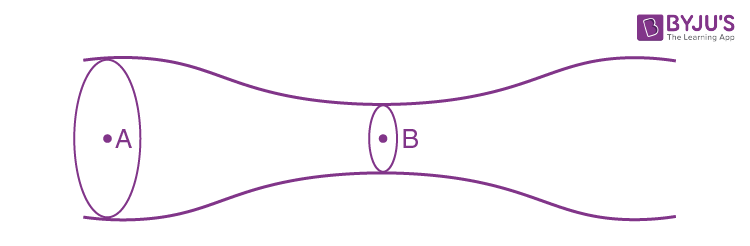a) 2720 cm
b) 2420 cm
c) 1810 cm
d) 3020 cm

Using equation of continuity

VA ×AreaA= VB ×AreaB

40VA=20VB

2VA = VB

Using Bernoulli’s equation

PA + ½ ?VA2 = ???? + ½ ??VB2

PA – PB = ½ ?? (VB2 – VA2 )

?P = ½ 1000( VB2 – VB2/4

= 500×3VB 2/4

VB = v(?P×4/1500)

= v(700×4/1500)

= v(28/15)m/s

Volume flow rate = VB×AreaB

= 20 × 100 ×v(28/15)cm3/s

= 2732.5 cm3/s

So, answer comes nearly 2720 cm3/s

Question 11. A screw gauge advances by 3 mm on main scale in 6 rotations. There are 50 divisions on circular scale. Find least count of screw gauge?
a) 0.01 cm
b) 0.001 cm
c) 0.001 mm
d) 0.02 mm

Pitch = 3/6 = 0.5 mm

Least count =Pitch / number of divisions

= 0.5mm/ 50

= 1100mm

= 0.01 mm

= 0.001 cm

Question 12. A telescope of aperture diameter 5 m is used to observe the moon from the earth. Distance between the moon and earth is 4 × 105 km. The minimum distance between two points on the moon’s surface which can be resolved using this telescope is close to (Wavelength of light is 5500 A° )
a) 60 m
b) 20 m
c) 600 m
d) 200 m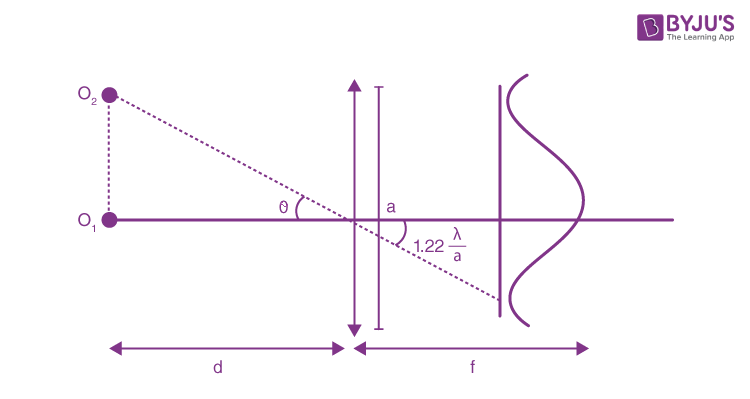Minimum angle for clear resolution,

?= 1.22 (?/a)

d = 1.22 (?/a)d

r = 4×108 m

Aperture, a = 5

= 5500 A0

Distance = r d

= 4×108 ×1.22 (5500×10-10/5)

= 53.68

Nearest option is 60m.

Question 13. Radiation with wavelength 6561 A° falls on a metal surface to produce photoelectrons. The electrons are made to enter a uniform magnetic field of 3×10-4 T. If the radius of largest circular path followed by electron is 10 mm, the work function of metal is close to;
a) 1.8 eV
b) 0.8 eV
c) 1.1 eV
d) 1.6 eV

From photoelectric equation,

hc/?=W+K.E max

Where, hc = 12400 eV A°

?12400/6561=W+K.E max

?1.89 eV=W+K.E max …(1)

Radius of charged particle moving in a magnetic field is given by

r = mv/qB

½ mv2 = K.Emax = eV

$$\begin{array}{l}r = \frac{\frac{\sqrt{2ev}}{m}\times m}{eB}= \frac{1}{B}\sqrt{\frac{2mv}{e}}\end{array}$$

?V=0.8 V

So, K.Emax=0.8 eV

Substituting in (1),

1.89 =W+0.8

i.e. W=1.1 eV (approx)

Question 14. Kinetic energy of the particle is ?? and it’s de–Broglie wavelength is ??. On increasing its K.E by ???, it’s new de–Broglie wavelength becomes ?/2. Then ??? is
a) 3E
b) E
c) 2E
d) 4E

?? = h/????

= hv(2??(????))

??? ? 1v????

??/??/2 = v(?????? / ??????

4?????? = ??????

???? = ?????? -??????

= 4??????-??????

= 3??????

= 3??

Question 15. A quantity is given by f = v(hc5/G), where c is speed of light, G is universal gravitational constant and h is the Planck’s constant. Dimension of f is that of;
a) area
b) energy
c) volume
d) momentum

hc = E?

[E] = [ML2T-2]

Therefore [hc] = [ML3T-2] [c] = [LT-1] [G] = [M-1 L3T-2]

v(hc5/G) = [ML2T-2]

The above dimension is of energy.

Question 16. A vessel of depth 2h is half filled with a liquid of refractive index v2 in upper half and with a liquid of refractive index 2v2 in lower half. The liquids are immiscible. The apparent depth of inner surface of the bottom of the vessel will be;
a) 3hv2/4
b) h/v2
c) h/3v2
d) h/2(v2+1)

Assume, air is present outside container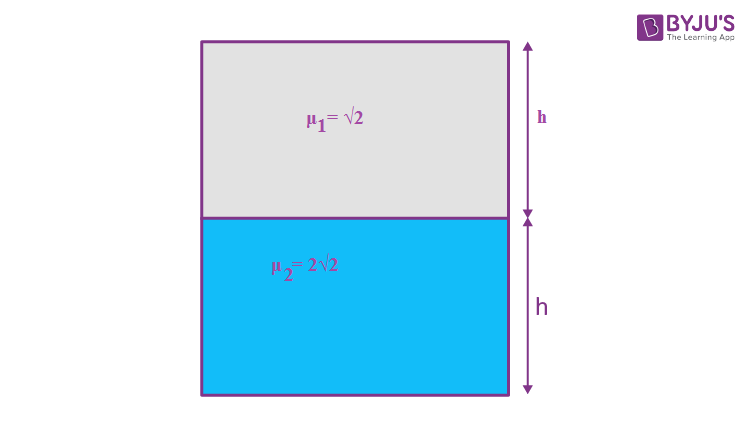Apparent height as seen from liquid 1 (having refractive index ??1 = v2 ) to liquid 2 (refractive index ??2 = 2v2)

D = h??1/??2 = h/2

Now, actual height perceived from air, h + h/2 = 3h/2

Therefore, apparent depth of bottom surface of the container (apparent depth as seen from air (having refractive index ??0 = 1) to liquid 1(having refractive index ??1= v2 )

= (3h/2)×(??0/ ??1 )

= (3h/2)×(1/v2)

= 3h/2v2

= 3v2h/4

Question 17. In the given circuit diagram, a wire is joining point B & C. Find the current in this wire;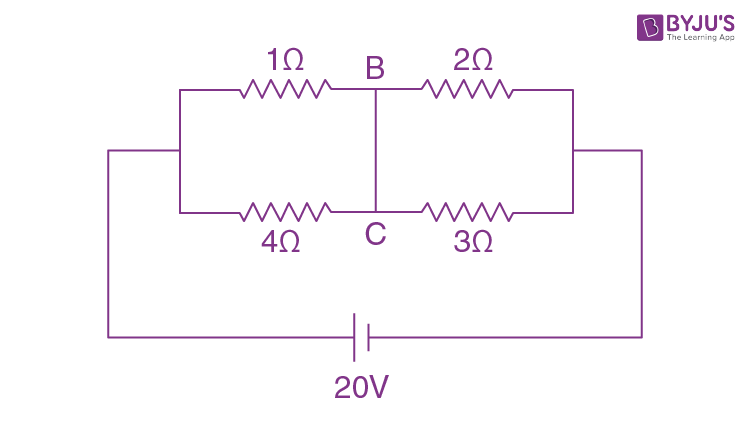a) 0.4 A
b) 2 A
c) 0
d) 4 A

Since resistance 1 O and 4 O are in parallel

???’ = 4×1/(4+1) = 4/5

Similarly we can find equivalent resistance (??”) for resistances 2 O and 3 O

???’’ = 6/5

And ??’ and ??” are in series

? ???????? = (4/5) + (6/5) = 2 O

So total current flowing in the circuit ‘??’ can be given as

?? = ??/???????? = 20/2 = 10A

Current will distribute in ratio opposite to resistance.

So, distribution will be as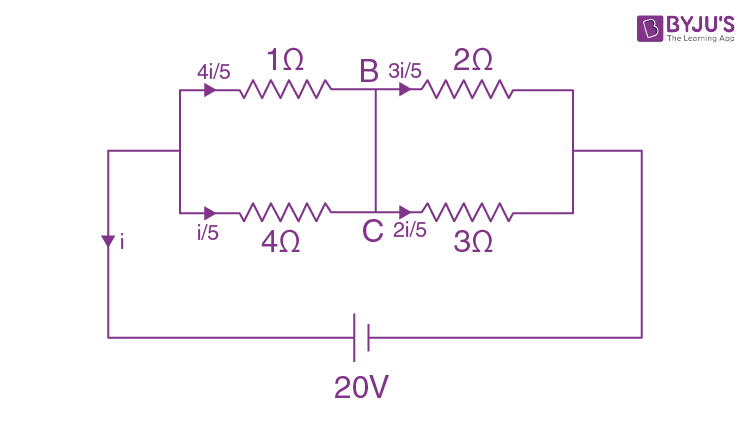So current in the branch BC will be ?? = (4??/5) – (3??/ 5)

= ??/5

= 10/ 5

= 2 A

Question 18. Two plane electromagnetic waves are moving in vacuum in whose electric field vectors are given by

$$\begin{array}{l}\vec{E_{1}} = E_{0}\hat{j} \cos (kx – \omega t)\end{array}$$
and
$$\begin{array}{l}\vec{E_{2}} = E_{0}\hat{k} \cos (ky – \omega t)\end{array}$$
. At t = 0 A charge q is at origin with velocity
$$\begin{array}{l}\vec{v} = 0.8c\hat{j}\end{array}$$
(?? is speed of light in vacuum). The instantaneous force on this charge (all data are in SI units)

a)

$$\begin{array}{l}qE_{0}(0.4\hat{i}-3\hat{j}+0.8\hat{k})\end{array}$$

b)
$$\begin{array}{l}qE_{0}(0.8\hat{i}+\hat{j}+0.2\hat{k})\end{array}$$

c)
$$\begin{array}{l}qE_{0}(0.8\hat{i}-\hat{j}+0.4\hat{k})\end{array}$$

d)
$$\begin{array}{l}qE_{0}(-0.8\hat{i}+\hat{j}+\hat{k})\end{array}$$

Given that the magnetic field vectors are:

$$\begin{array}{l}\vec{E_{1}} = E_{0}\hat{j} \cos (kx – \omega t)\end{array}$$
$$\begin{array}{l}\vec{E_{2}} = E_{0}\hat{k} \cos (ky – \omega t)\end{array}$$

Since, the variation of vectors E1 and E2 are along x and y respectively.

Therefore, direction of propagation of vectors E1 and E2 will be along x and y respectively. Since

$$\begin{array}{l}\vec{E}\times \vec{B}\end{array}$$
gives direction of propagation and E0/B0 = c and variation of magnetic field will be same to magnetic field.

So, the magnetic field vectors of the electromagnetic wave are given by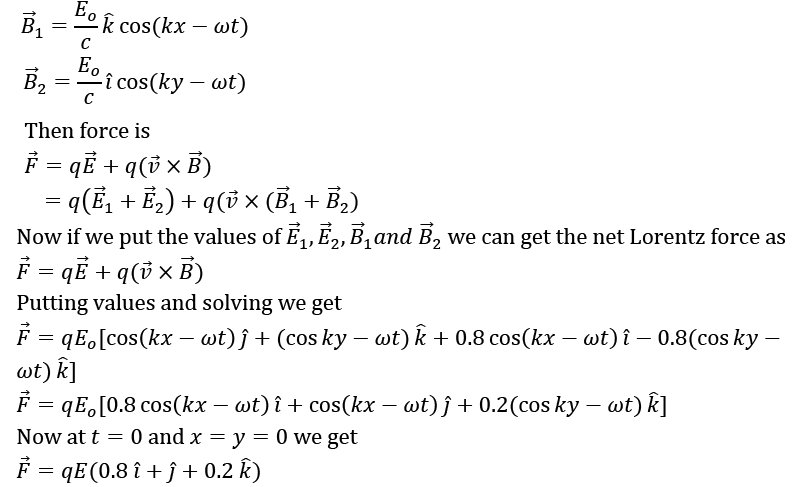Question 19.Consider two ideal diatomic gases A and B at some temperature T. Molecules of the gas A are rigid, and have a mass m. Molecules of the gas B have an additional vibration mode and have a mass m/4 . The ratio of molar specific heat at constant volume of gas A and B is;
a) 7/9
b) 5/9
c) 3/5
d) 5/7

We know that,

Molar heat capacity at constant volume, ???? = ????/2 (Where f is degree of freedom)

Since, A is diatomic and rigid, degree of freedom for A is 5

Therefore, Molar heat capacity of A at constant volume (Cv)A = 5R/2

Since, B is diatomic and has extra degree of freedom because of vibration; degree of freedom for B is 5 + 2×1= 7 (1 vibration for each atom).

Therefore, Molar heat capacity of B at constant volume (CV)B = 7R/2

Ratio of molar specific heat of A and B = (CV)A / (CV)B = 5/7

Question 20. A charged particle of mass ‘m’ and charge ‘q’ is moving under the influence of uniform electric field
$$\begin{array}{l}E\hat{i}\end{array}$$
and a uniform magnetic field
$$\begin{array}{l}B\hat{k}\end{array}$$
follow a trajectory from P to Q as shown in figure. The velocities at P and Q are respectively
$$\begin{array}{l}v\hat{i}\end{array}$$
and
$$\begin{array}{l}-2v\hat{j}\end{array}$$
. Then which of the following statements (A, B, C, D) are correct? (Trajectory shown is schematic and not to scale)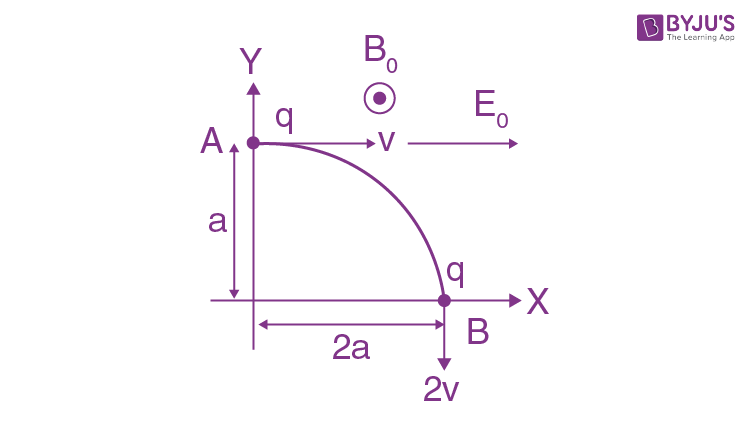A. Magnitude of electric field

$$\begin{array}{l}\vec{E} = \frac{3}{4}\left ( \frac{mv^{2}}{qa} \right )\end{array}$$

B. Rate of work done by electric field at P is

$$\begin{array}{l}\frac{3}{4}\left ( \frac{mv^{3}}{a} \right )\end{array}$$

C. Rate of work done by both fields at Q is zero.

D. The difference between the magnitude of angular momentum of the particle at P and Q is 2mva.

a) A, C and D are correct
b) A, B and C are correct
c) A, B. C and D are correct
d) B, C and D are correct

Considering statement A

Let, Net work done by magnetic field be WB and net work done by electric field be WE.

By Work-Energy theorem

W??+???? = (1/2)??(2??)2 – (1/2) mv2

? 0+??????2?? = (3/2) ????2

???? = (3/4) ????2 /????

So, statement A is correct

Now, considering statement B

Rate of work done at P = Power of electric force

= ????????

= (3/4) ????3 /??

So, statement B is correct

Now, considering statement C

At Q,

Vector E perpendicular to vector v.

Vector B perpendicular to vector v.

So, dw/dt = 0 for both forces

$$\begin{array}{l}\frac{dw}{dt}= q(\vec{E}+\vec{v}\times \vec{B}).\vec{v}\end{array}$$

So, statement C is correct

Now, considering statement D

Angular momentum should be defined about a point which is not given in question but let’s find angular momentum about origin.

Change in magnitude of angular momentum of the particle at P and Q about origin

$$\begin{array}{l}\Delta \vec{L} = \Delta \vec{L_{P}}-\Delta \vec{L_{Q}}\end{array}$$
$$\begin{array}{l}\Delta \vec{L_{Q}}= m (2v)(2a)\end{array}$$
$$\begin{array}{l}\Delta \vec{L_{P}}= m (v)(a)\end{array}$$

Hence, ??? = 3mva

So, statement D is wrong.

Question 21. In a fluorescent lamp choke (a small transformer) 100 V of reversible voltage is produced when choke changes current in from 0.25 A to 0 A in 0.025 ms. The self-inductance of choke (in mH) is estimated to be;

Fluorescent lamp choke will behave as an inductor

By using faraday law to write induced emf,

? -L dI/dt = 0

100 = L(0.25)×103/0.025

L = 100×10-4 H

= 10 mH

Question 22.A wire of length l = 0.3 m and area of cross section 10–2 cm2 and breaking stress 4.8×107 N/m2 is attached with block of mass 10 kg. Find the maximum possible value of angular velocity (??????/??) with which block can be moved in a circle with string fixed at one end.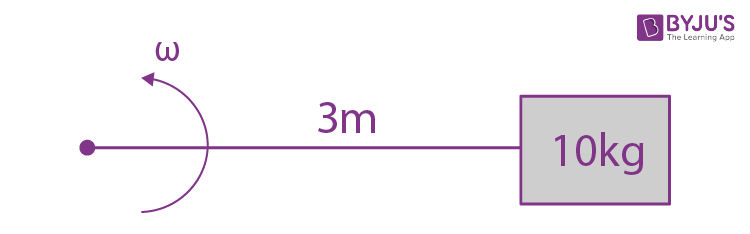Breaking stress ?? = T/A

T = ????2l

? ?? = ????2l/??

? ??2 = As/mI

= (4.8×107×10-6)/ 10×0.3 = 16

??? = 4 ??????/??

Question 23. The distance x covered by a particle in one dimension motion varies as with time ?? as x2 = at2 + 2bt + c, where a, b, c are constants. Acceleration of particle depend on x as x–n , the value of n is;

Let v be velocity,a be the acceleration then,

x2 = at2 + 2bt + c

2xv = 2at + 2b

xv = at + b ..(i)

v = (at + b)/x

Now, differentiating equation (i)

v2 + ax = a

ax = a – (at+b)2/x2

a= a(at2 +2bt+c)-(at+b2)/x3

a= ac – b2/x3

a ? x-3

Question 24. A rod of length 1 m pivoted at one end is released from rest when it makes 30° from the horizontal as shown in the figure below.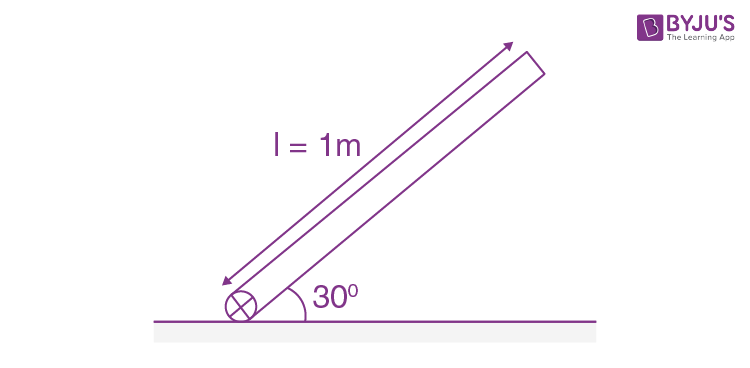If ? of rod is v?? at the moment it hits the ground, then find n.

By using conservation of energy,

mg (??/2)sin30° = (½)(m??2/3)?2

On solving ?2 = 15

??=v15

Therefore, n = 15.

Question 25. In the given circuit both diodes are ideal having zero forward resistance and built-in potential of 0.7 V. Find the potential of point E in volts.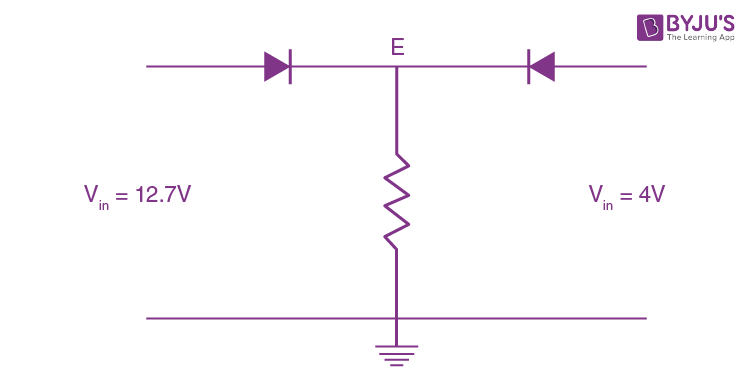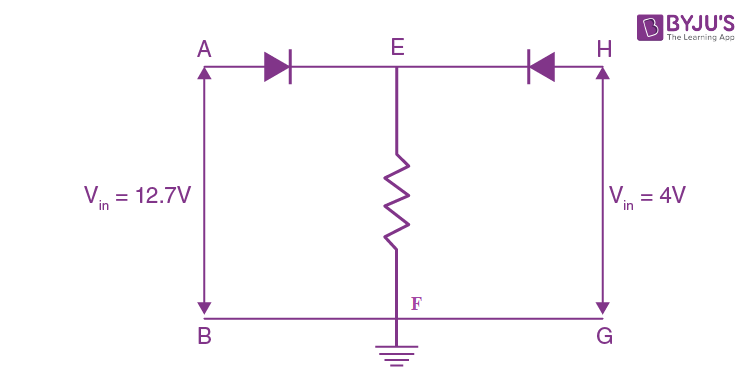We have to apply nodal analysis on both left and right side and check what can be voltage at E. For nodal analysis, voltage at B, F and G will be 0 volts and voltage at A will be 12.7 volt and voltage at H will be 4 volts.

If, we apply Nodal from right side, voltage at E will be 12 volt (diode between A and E will be forward biased). Now voltage at E is 12 volt and voltage at H is 4 volt and since, diode between E and H is reversed biased and any difference of voltage is possible across reverse biased. So, this is possible.

If, we apply Nodal from left side, voltage at E will be 3.3 volt (diode between E and H will be forward biased). Now voltage at E is 3.3 volt and voltage at A is 12 volt and since, diode between E and A is forward biased and in forward biased difference of voltage of 0.7 volt is allowable. So, this case is not possible. Therefore current will also not flow through GH. Hence, VE = 12 V.